# AFOQT: Quadratic Equations Chapter Exam

Exam Instructions:

Choose your answers to the questions and click 'Next' to see the next set of questions. You can skip questions if you would like and come back to them later with the yellow "Go To First Skipped Question" button. When you have completed the practice exam, a green submit button will appear. Click it to see your results. Good luck!

### Page 1

#### Question 1 1. In order to complete the square on the following equation, what value would you add to both sides?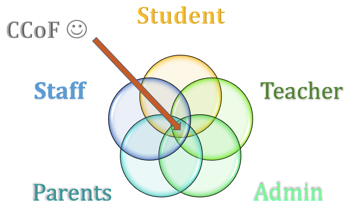#### Question 2 2. When completing the square on the quadratic equation listed below, how is c calculated?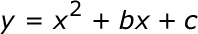#### Question 3 3. Find the value for c that needs to be added to both sides and rewrite the equation in the vertex form.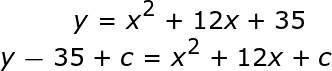### Page 2

#### Question 9 9. What would the first step be in completing the square of the following equation?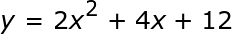### Page 3

#### Question 13 13. Expressing the equation below in the vertex form will give a value of 'h' = _____ .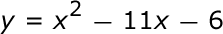#### Question 14 14. Solve the equation below.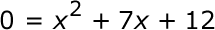### Page 5

#### Question 23 23. Solve the equation below.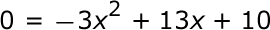#### Question 24 24. Rewrite the following equation in the vertex form.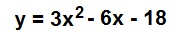### Page 6

#### Question 30 30. If the equation below told you a ball was 'h' feet in the air after 't' seconds, what would be the first step to finding out when it hit the ground?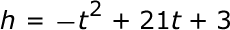#### AFOQT: Quadratic Equations Chapter Exam Instructions

Choose your answers to the questions and click 'Next' to see the next set of questions. You can skip questions if you would like and come back to them later with the yellow "Go To First Skipped Question" button. When you have completed the practice exam, a green submit button will appear. Click it to see your results. Good luck!

Support